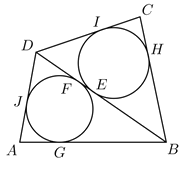Mathematical and Physical Journal
for High Schools
Issued by the MATFUND Foundation
 Already signed up? New to KöMaL?

#Problem K. 566. (December 2017)

K. 566. The lengths of the sides of a quadrilateral $\displaystyle ABCD$ are $\displaystyle AB = 8$ cm, $\displaystyle BC= 7$ cm, $\displaystyle CD = 6$ cm and $\displaystyle DA = 5$ cm. The inscribed circles of triangles $\displaystyle BCD$ and $\displaystyle ABD$ touch diagonal $\displaystyle BD$ at points $\displaystyle E$ and $\displaystyle F$, respectively. What is the length of the line segment $\displaystyle EF$?

(6 pont)

Deadline expired on January 10, 2018.

Sorry, the solution is available only in Hungarian. Google translation

Megoldás. Egy körhöz egy külső pontból húzott érintőszakaszok hossza egyenlő.Az $\displaystyle ABD$ háromszögben $\displaystyle AG = AJ$, $\displaystyle GB = BF = 8 – AG$, valamint $\displaystyle JD = DF = 5-AJ=5 – AG$, így $\displaystyle DB = (5– AG) + (8 – AG) = 13 – 2AG$.

A $\displaystyle BCD$ háromszögben hasonlóan $\displaystyle DB = (7– CH) + (6 – CH) = 13 – 2CH$, így $\displaystyle AG = CH$.

$\displaystyle FE = DB – DF – BE = 13 – 2AG – (5 – AG) – (7 – CH) = 1$.

### Statistics:

 75 students sent a solution. 6 points: 62 students. 5 points: 2 students. 2 points: 4 students. 1 point: 1 student. 0 point: 6 students.

Problems in Mathematics of KöMaL, December 2017